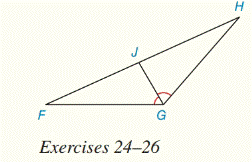Chapter 5.CR, Problem 26CRElementary Geometry For College St...

7th Edition
Alexander + 2 others
ISBN: 9781337614085

Solutions

Chapter
SectionElementary Geometry For College St...

7th Edition
Alexander + 2 others
ISBN: 9781337614085
Textbook Problem

For Review Exercises 24 to 26, G J → bisects ∠ F G HGiven: F G = 8 , H G = 12 , F H = 15 Find FJ.

To determine

To find:

FJ.

Explanation

Given:

FG=8,HG=12,FH=15,FH=15 and

Calculation:

Let FJ=x

Then, JH=FHx=15x

Using, “If a ray bisects one angle of a triangle, then it divides the opposite side into segments whose lengths are proportional to the lengths of the two sides that from the bisected angle”.

So,

FJFG=JHGH

Still sussing out bartleby?

Check out a sample textbook solution.

See a sample solution

The Solution to Your Study Problems

Bartleby provides explanations to thousands of textbook problems written by our experts, many with advanced degrees!

Get Started

Find more solutions based on key concepts